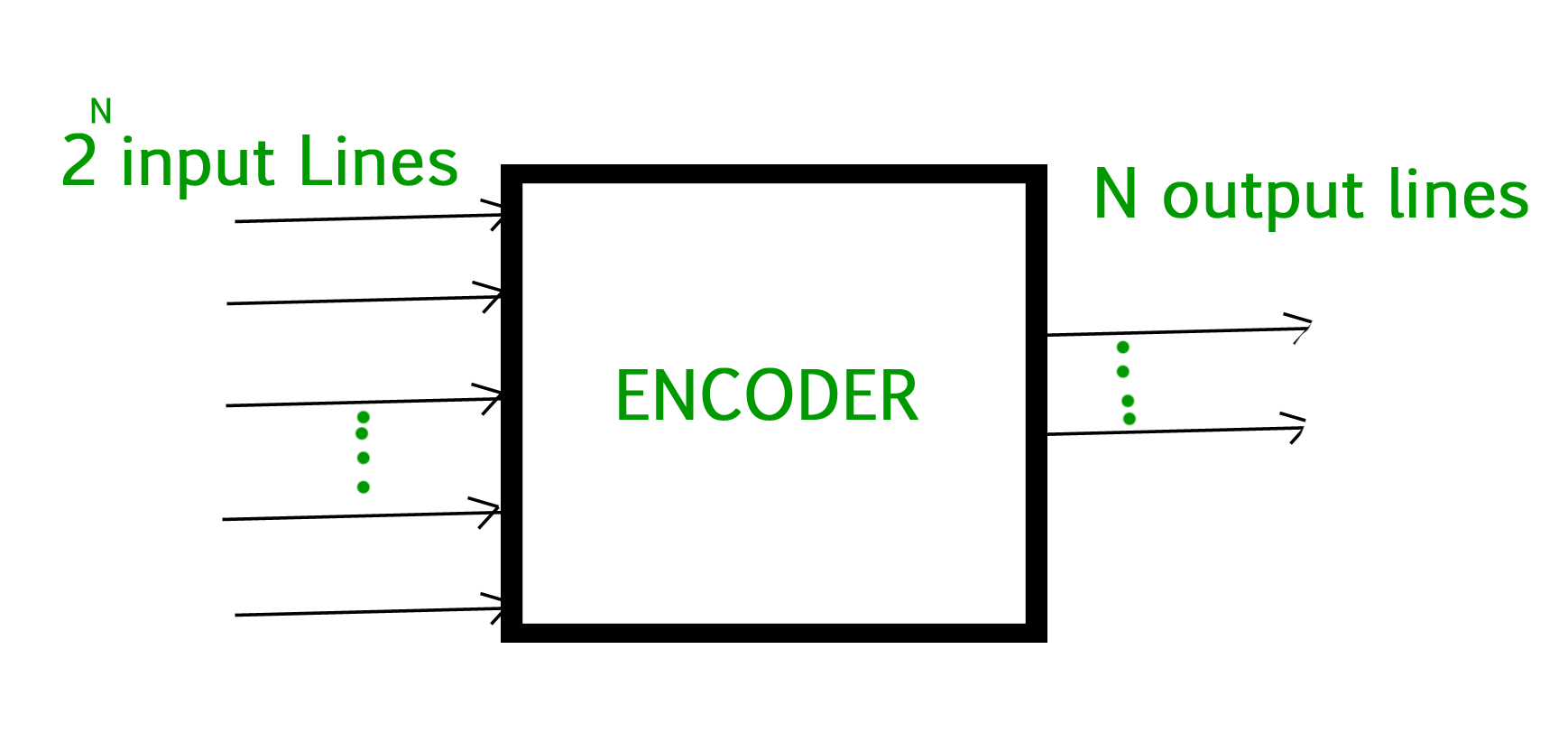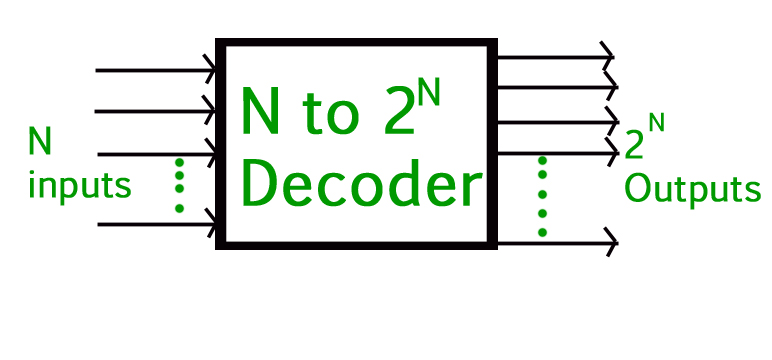# Difference between Encoder and Decoder

• Difficulty Level : Medium
• Last Updated : 22 Oct, 2021

Combinational logic is the concept in which two or more input state define one or more output state. Encoder and Decoder are the combinational logic circuits. In which we implement combinational logic with the help of boolean algebra.

To encode something is to convert an unambiguous piece of information into a form of code that is not so clearly understood and the device which performs this operation is termed ad Encoder.

1. Encoder :
An Encoder is a device that converts the active data signal into a coded message format or it is a device that converts analogue signal to digital signals. It is a combinational circuit, that converts binary information in the form of a 2N input lines into N output lines which represent N bit code for the input. When an input signal is applied to an encoder then logic circuitry involved within it converts that particular input into coded binary output.To decode is to perform the reverse operation :converting a code back into an unambiguous form code and the device which perform this operation is termed as Decoder.

2. Decoder :
A decoder is also a combinational circuit as encoder but its operation is exactly reverse as that of the encoder. A decoder is a device that generates the original signal as output from the coded input signal and converts n lines of input into 2n lines of output. An AND gate can be used as the basic decoding element because it produces a high output only when all inputs are high.Difference between encoder and decoder :

My Personal Notes arrow_drop_up